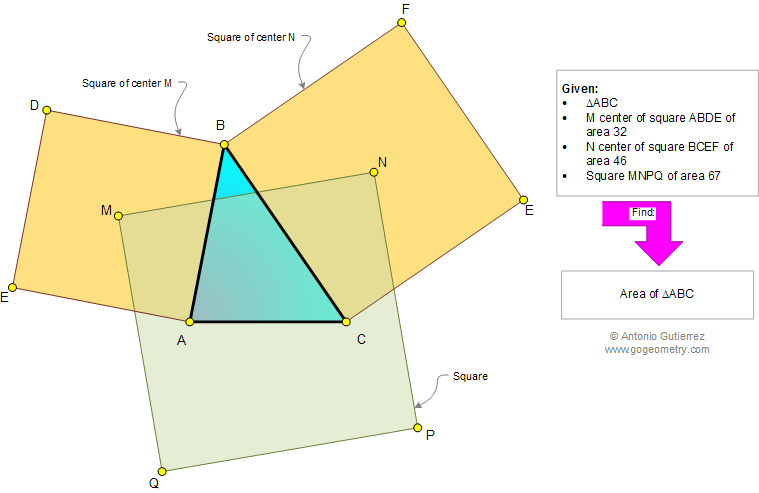# Online Geometry Problem 881: Triangle, three Squares, Centers, Areas. Level: High School, Honors Geometry, College, Mathematics Education

 The drawing shows a triangle ABC, M is the center of the square ABCD of area 32, N is the center of the square BCEF of area 46, and MNPQ is a square of area 67. Find the area of triangle ABC.Geometry problem solving is one of the most challenging skills for students to learn. When a problem requires auxiliary construction, the difficulty of the problem increases drastically, perhaps because deciding which construction to make is an ill-structured problem. By “construction,” we mean adding geometric figures (points, lines, planes) to a problem figure that wasn’t mentioned as "given."
 Home | Search | Geometry | Problems | All Problems | Open Problems | Visual Index | 10 Problems | Problems Art Gallery | Art | 881-890 | Triangle | Square | Area | Square area | Solution / comment | By Antonio Gutierrez